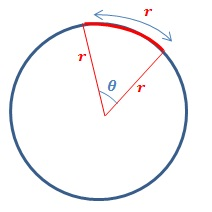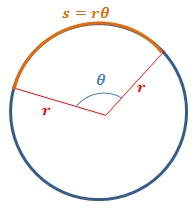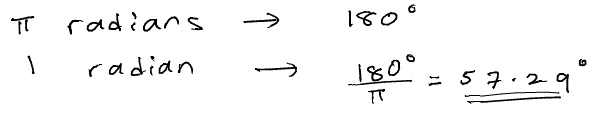# How to Find Angular Speed

In this article, we will look at how to find angular speed. Before we do so, it’s important to become familiar with using radians, which is a unit that we use to measure angles.

## Radian Measure of an Angle

In everyday situations, we are used to measuring angles using degrees. We divide a circle into 360 portions, and we define one degree to be the angle subtended by an arc, whose length is$\frac{1}{360}$ of the circumference of the circle.

But why the number 360? 360 is a number that is easily divisible by many whole numbers, so often, calculations involving angles measured in degrees can be simplified to simpler fractions. However, there’s no real physical reason for dividing a circle into 360 parts. In fact, using degrees to measure angles in calculus problems can become cumbersome. It is much better to use a unit to measure angles that is defined by the properties of a circle alone.

Radians are such a unit. In more advanced physics and mathematics, problems involving angles are done using radians most of the time. By default, angle calculations in spreadsheet software are also given in radians. Scientific calculators also have a radian mode that allows us to do calculations directly using radians.

So what is a radian? A radian is defined as the angle subtended by an arc whose length is equal to the length of the circle’s radius.This definition gives an interesting property. In a circle with radius$r$, the length$s$ of an arc that subtends an angle of$\theta$ radians is given by,$s=r\theta$Radians are a dimensonless unit, because it is a ratio of two lengths. The units from each of the lengths cancel out when we take the ratio.

Consider a semicircle. The angle subtended by a semicirce is 180o. Since the circumference of a circle is given by$2\pi r$, the arc length of the semicircle is$\pi r$. Because$s=r\theta$, we have$\pi r=r \theta$. This means that an angle of 180o is equivalent to$\pi$ radians.

We can use this conversion factor to convert any angle given in degrees to radians, and vice versa.$\mathrm{angle\: in\: radians=\frac{angle\: in\: degrees}{180^{o}}\times\pi}$

Example 1

Find the size of an angle of 1 radian in degrees.## How to Find Angular Speed

If an object moving in a circle at a constant speed sweeps through an angle of$\Delta \theta$ in a time of$\Delta t$, the angular speed$\omega$ of the object is defined as,$\omega=\frac{\Delta \theta}{\Delta t}$

The time taken by an object moving in a circular path to move through one full cycle is called the period,$T$. In other words, the object moves through an angle of 360o, i.e.$2\pi$ radians, during this time. Using the previous equation, then, we can write:$\omega=\frac{2\pi}{T}$

Often, angular speeds of objects are given in terms of the number of revolutions per minute (rpm). To do calculations, sometimes it is necessary to convert this to value in radians per second. To do this, we use the fact that one revolution is equal to 360o.

Frequency$f$ is the total number of revolutions per unit time. It is defined,$f=\frac{1}{T}$

and has units hertz (Hz). 1 revolution per second = 1 Hz.

Since$\omega =\frac{2\pi}{T}$,$\omega =2\pi f$

### Example 2

A dental drill rotates at a speed of 200 000 rpm. Find its angular speed in radians per second.

## How to Find the Speed of an Object in Circular Motion

The angular speed gives the angle that an object moving in a circular path sweeps out per second. The speed of the object (sometimes referred to as “linear speed”) is still the distance that the object travels per unit time. If the object travels through a length$s$ along the circumference of the circle during a time$t$, then the speed$v$ of the object is,$v=\frac{s}{t}$

Since$s=r\theta$, we can write,$v=r\frac{\theta}{t}$

Since$\frac{\theta}{t}=\omega$, we can write$v=r \omega$

This is the relationship between an object’s angular speed$\omega$ and its speed,$v$.

At any given moment, the direction of the paticle’s speed is at a tangent to the circular path. If you rotate something in a circle and suddenly let go, the object would fly off at a tangent to the circle. For this reason, speed of the object is also referred to as tangential speed.

### Example 3

The London Eye is one of the largest Ferris wheels on Earth. It has a diameter of 120 m, and rotates at a rate of about 1 complete rotation per 30 minutes. Find the speed of a passenger traveling on it.

## Angular Speed Calculation – Additional Examples

### Example 4

A DVD player makes a DVD spin at 1600 rpm. Find the period of the DVD’s rotation.

Here, there is no need to convert rpm to radians per second. The period can be calculated directly.

### Example 5

The second hand of a clock goes around smoothly in a circle. An ant is sitting on the edge of the hand. If the ant is going around at a speed of 2 cm s-1, find the length of the second hand.

Note that in the above calculation, it was not necessary to convert the speed to metres per second. Since we kept the units as centimetres, our answer is also in centimetres.